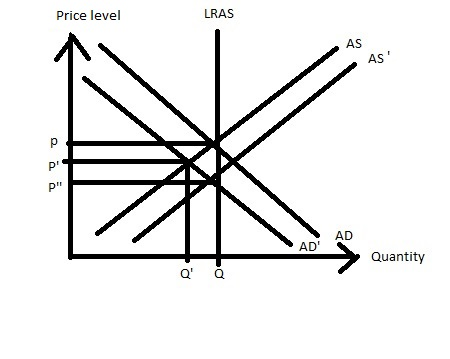# Starting from long-run equilibrium in the dynamic model of aggregate demand and aggregae supply,...

## Question:

Starting from long-run equilibrium in the dynamic model of aggregate demand and aggregae supply, a one-period positive supply shock causes output to:

A) remain above the natural level for only one period.

B) remain above the natural level for more than one period.

C) remain below the natural level for only one period.

D) remain below the natural level for more than one period.

## Aggregate supply:

In the long run, the economy is at its full-employment level of output where the actual GDP is equal to potential GDP such that the aggregate supply curve is vertical.

## Answer and Explanation:

In the long run, a one-period positive supply shock results in an increase in the aggregate supply curve and at the same time the aggregate demand curve shifts leftward which decreases the price level and output remains the same or full employment is achieved.So, option (D) remain below the natural level for more than one period is correct.

#### Learn more about this topic:Aggregate Supply and Aggregate Demand (AS-AD) Model

from Economics 102: Macroeconomics

Chapter 7 / Lesson 3
58K